#Algebra 1 Worksheets

## Exponents Worksheets

Here is a graphic preview for all of the Exponents Worksheets. You can select different variables to customize these Exponents Worksheets for your needs. The Exponents Worksheets are randomly created and will never repeat so you have an endless supply of quality Exponents Worksheets to use in the classroom or at home. We have evaluating exponents functions, graphing exponents, properties of exponents, writing numbers in scientific notation, and operations with scientific notation.

Our Exponents Worksheets are free to download, easy to use, and very flexible.

These Exponents Worksheets are a good resource for students in the 5th Grade through the 8th Grade.

## Quick Link for All Exponents Worksheets

Click the image to be taken to that Exponents Worksheets.

##### Exponents PropertiesHandout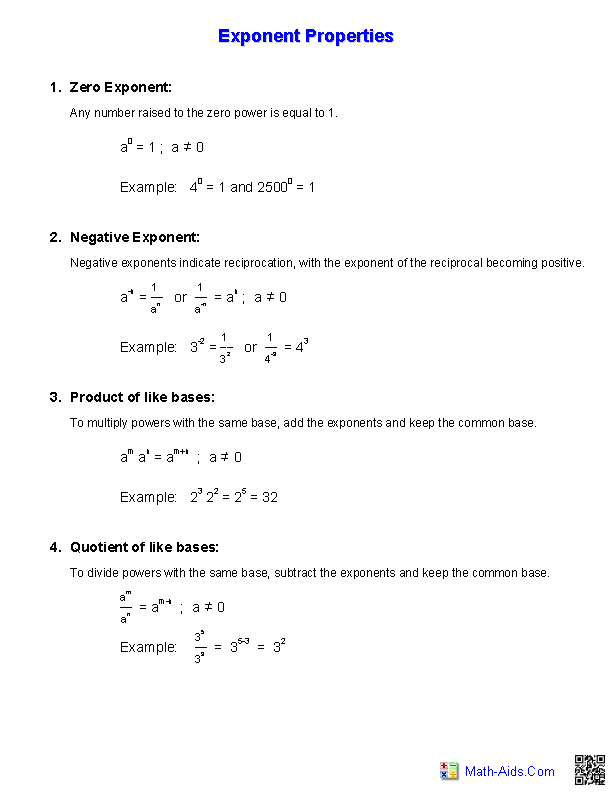##### Evaluating ExponentialFunctions Worksheets##### Graphing ExponentialFunctions Worksheets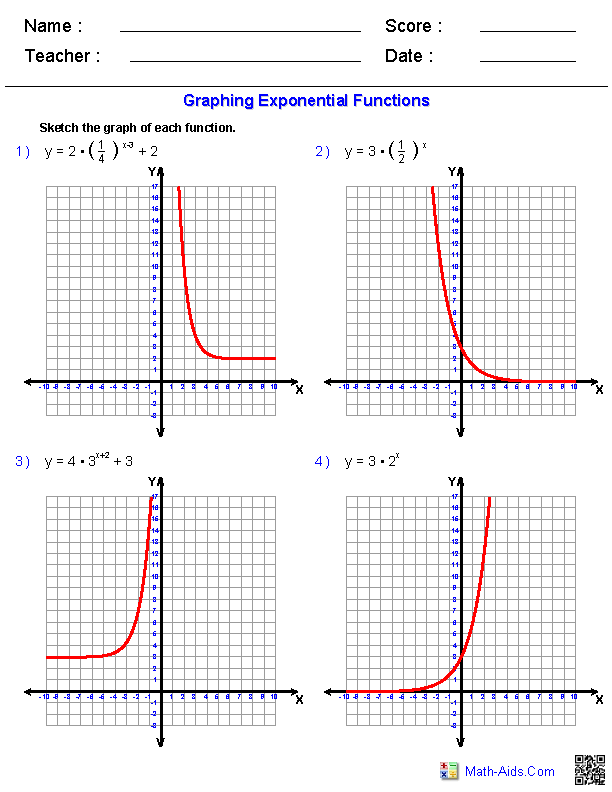##### Exponents withMultiplication Worksheets##### Exponents withDivision Worksheets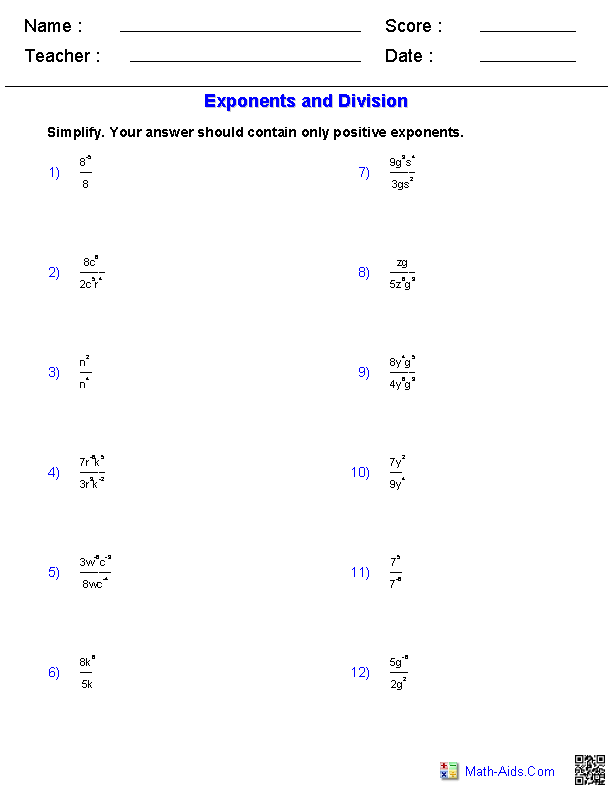##### Exponents with Multiplicationand Division Worksheets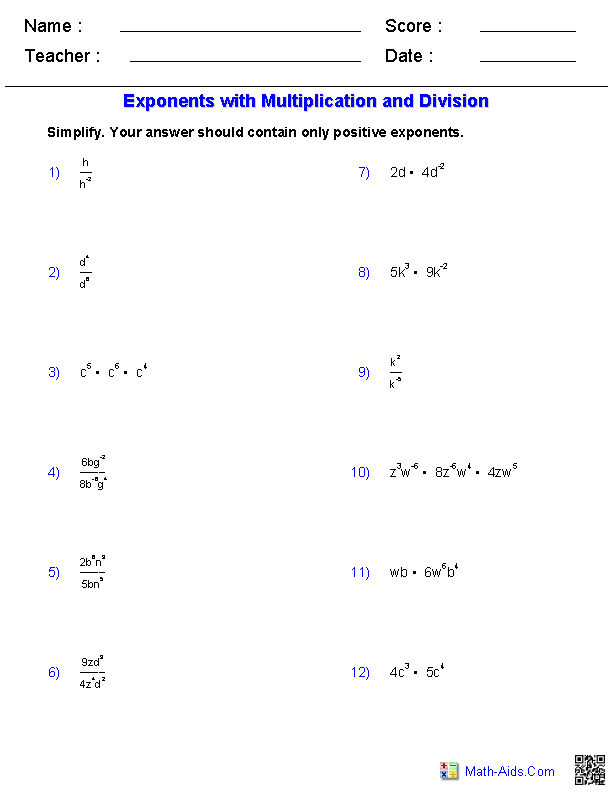##### Powers ofProducts Worksheets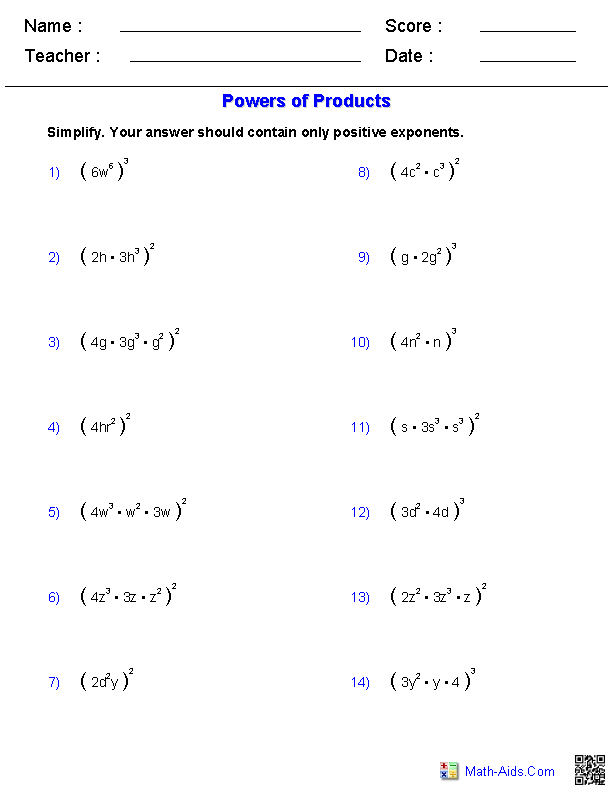##### Powers ofQuotients Worksheets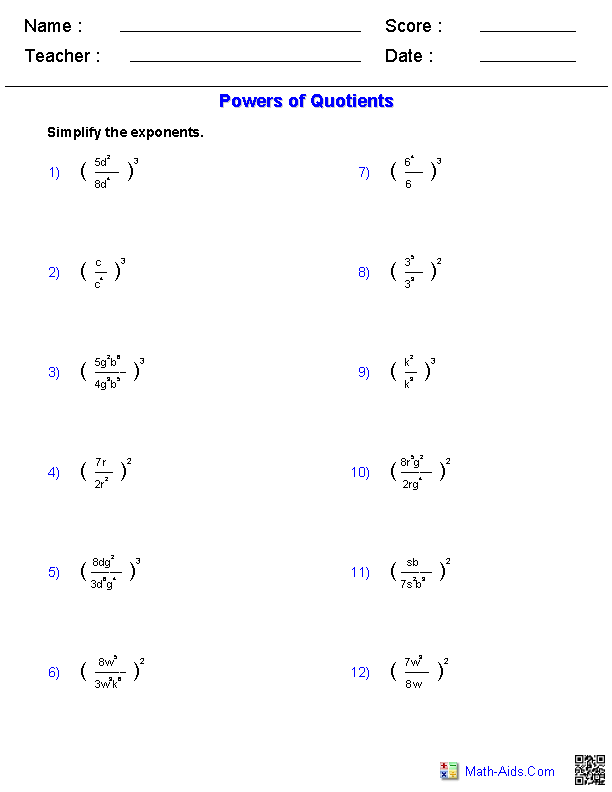##### Powers of Productsand Quotients Worksheets##### Operations withExponents Worksheets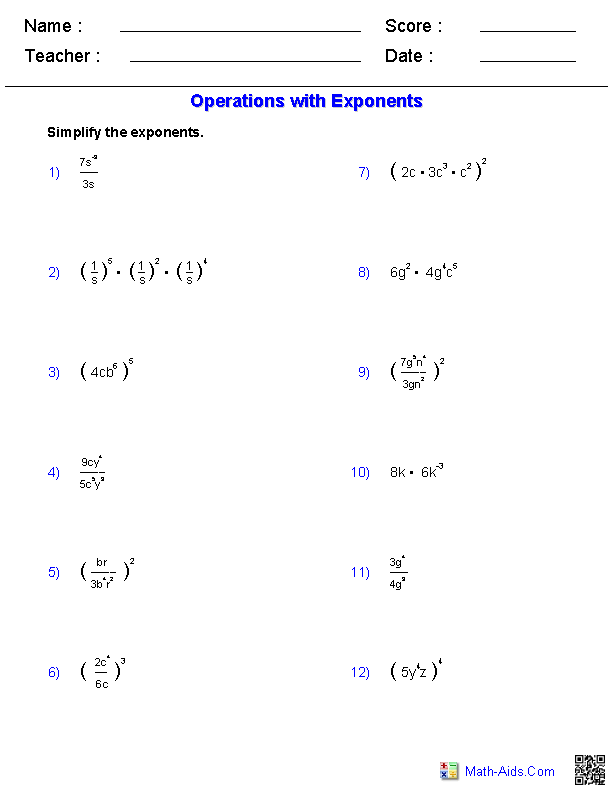##### Writing Numbersin Scientific Notation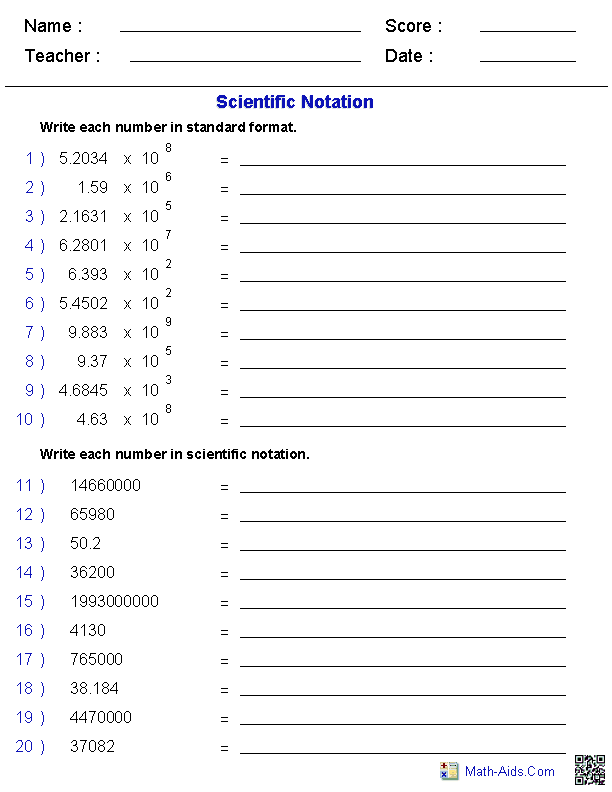##### Operations withScientific Notation Worksheets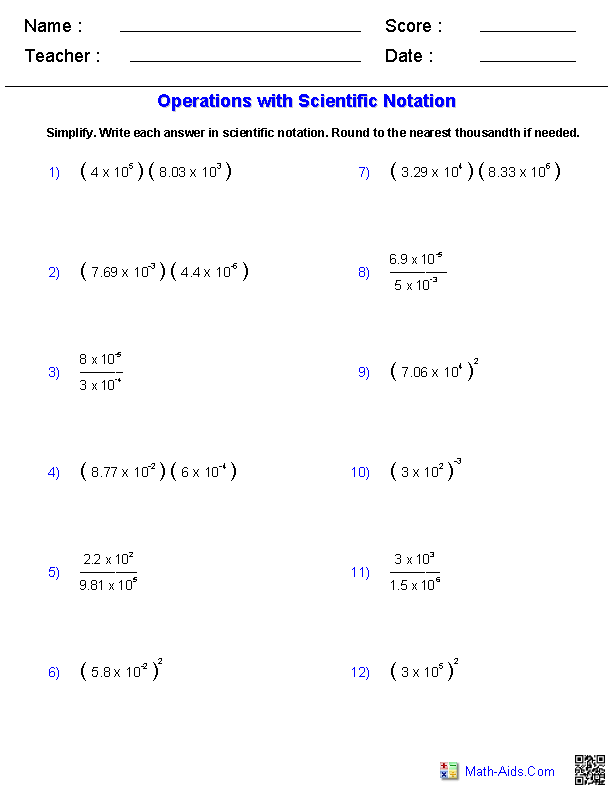Recommended Videos

## Detailed Description for All Exponents Worksheets

Exponents Properties Handout
These Algebra 1 - Exponents Worksheets will produce a handout to define and give examples for the different properties of exponents. These Exponents Worksheets are a good resource for students in the 5th Grade through the 8th Grade.

Evaluating Exponential Functions Worksheets
These Algebra 1 - Exponents Worksheets produces problems for evaluating Exponential Functions. You may select the problems to contain only positive, negative or a mixture of different exponents. These Exponents Worksheets are a good resource for students in the 5th Grade through the 8th Grade.

Graphing Exponential Functions Worksheets
This Algebra 1 Graphing Exponential Functions worksheets will give you exponent functions to graph. You may choose to graph an equation or write an equation from a graph. These Exponents Worksheets are a good resource for students in the 5th Grade through the 8th Grade.

Exponents with Multiplication Worksheets
These Algebra 1 - Exponents Worksheets produces problems for working with Exponents with Multiplication. You may select the problems to contain only positive, negative or a mixture of different exponents. These Exponents Worksheets are a good resource for students in the 5th Grade through the 8th Grade.

Exponents with Division Worksheets
These Algebra 1 - Exponents Worksheets produces problems for working with Exponents with Division. You may select the problems to contain only positive, negative or a mixture of different exponents. These Exponents Worksheets are a good resource for students in the 5th Grade through the 8th Grade.

Exponents with Multiplication and Division Worksheets
These Algebra 1 - Exponents Worksheet produces problems for working with Exponents with Multiplication and Division. You may select the problems to contain only positive, negative or a mixture of different exponents. These Exponents Worksheets are a good resource for students in the 5th Grade through the 8th Grade.

Powers of Products Worksheets
These Algebra 1 - Exponents Worksheet produces problems for working with products to a power. You may select the type of problems to use, and this worksheet produces fourteen problems per page. These Exponents Worksheets are a good resource for students in the 5th Grade through the 8th Grade.

Powers of Quotients Worksheets
These Algebra 1 - Exponents Worksheet produces problems for working with quotients to a power. You may select the type of problems to use, and this worksheet produces 12 problems per page. These Exponents Worksheets are a good resource for students in the 5th Grade through the 8th Grade.

Powers of Products and Quotients Worksheets
These Algebra 1 - Exponents Worksheet produces problems for working with products and quotients to a power. You may select the type of problems to use, and this worksheet produces 12 problems per page. These Exponents Worksheets are a good resource for students in the 5th Grade through the 8th Grade.

Operations with Exponents Worksheets
This Algebra 1 - Exponents Worksheet produces problems for working with different operations with exponents. You may select from exponents with multiplication or division and products or quotients to a power. This worksheet produces 12 problems per page. These Exponents Worksheets are a good resource for students in the 5th Grade through the 8th Grade.

Writing Numbers in Scientific Notation Worksheets
These Algebra 1 - Exponents Worksheet are great for teaching students to read and write numbers in scientific notation. The exponents for the scientific notation problems may be positive, negative, or both. You may also include a zero exponent by checking that box. These Exponents Worksheets are a good resource for students in the 5th Grade through the 8th Grade.

Operations with Scientific Notation Worksheets
This Algebra 1 - Exponents Worksheet produces problems for working with different operations with Scientific Notation. You may select problems with multiplication, division, or products to a power. This worksheet produces 12 problems per page. These Exponents Worksheets are a good resource for students in the 5th Grade through the 8th Grade.